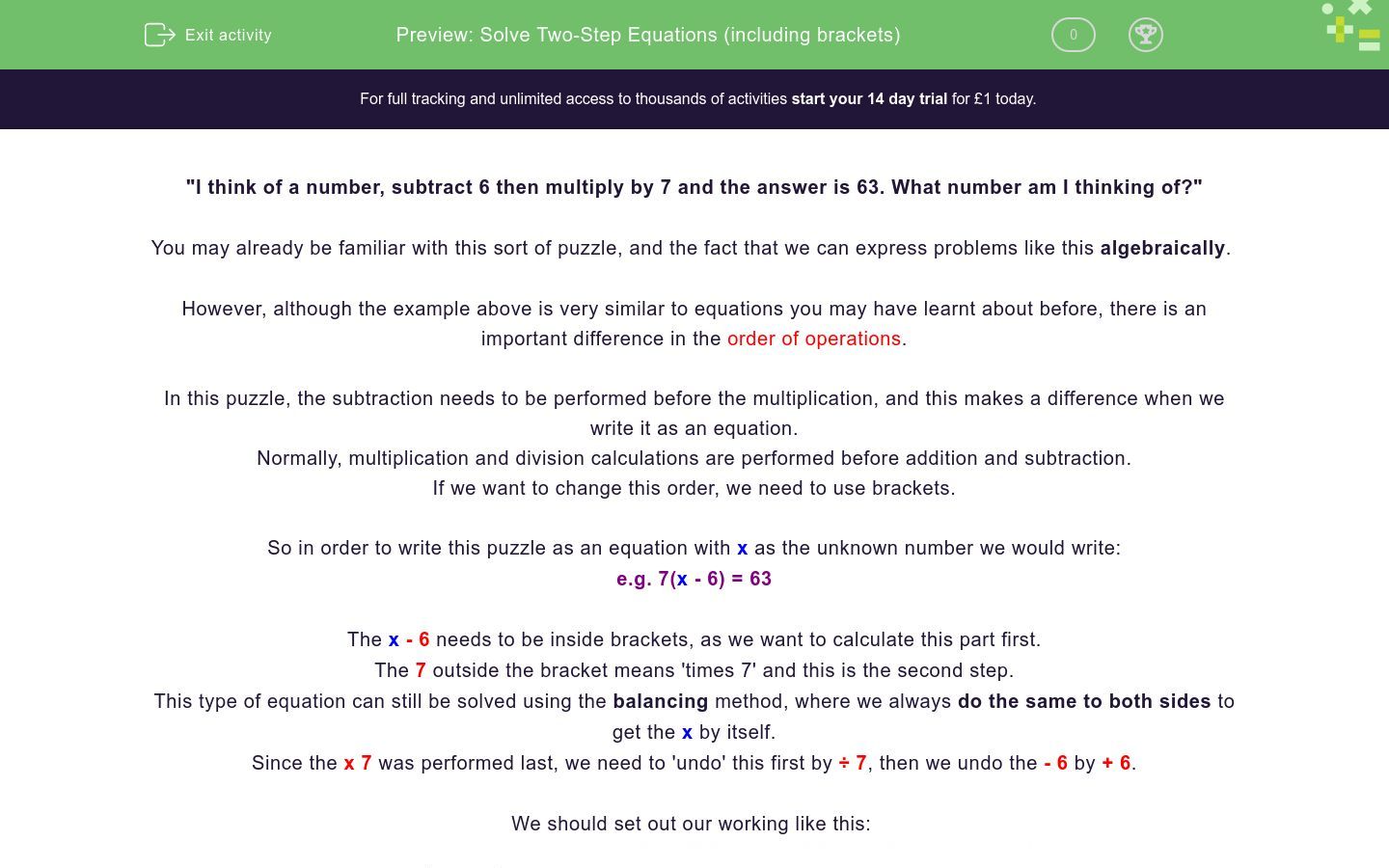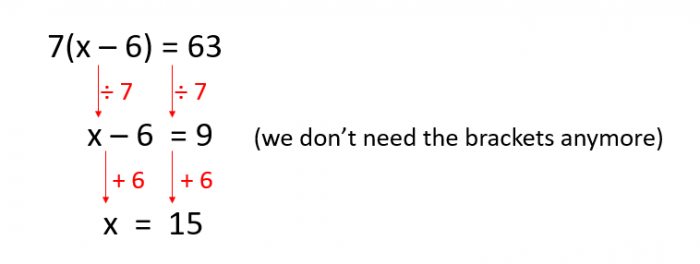# Solve Two-Step Equations (including brackets)

In this worksheet, students will learn how to solve two-step equations by finding inverse operations, plus performing them in the correct order, to reach accurate answers.Key stage:  KS 4

GCSE Subjects:   Maths

GCSE Boards:   Pearson Edexcel, OCR, Eduqas, AQA,

Curriculum topic:   Algebra

Curriculum subtopic:   Solving Equations and Inequalities Algebraic Equations

Difficulty level:#### Worksheet Overview

"I think of a number, subtract 6 then multiply by 7 and the answer is 63. What number am I thinking of?"

You may already be familiar with this sort of puzzle, and the fact that we can express problems like this algebraically

However, although the example above is very similar to equations you may have learnt about before, there is an important difference in the order of operations.

In this puzzle, the subtraction needs to be performed before the multiplication, and this makes a difference when we write it as an equation.

Normally, multiplication and division calculations are performed before addition and subtraction.

If we want to change this order, we need to use brackets.

So in order to write this puzzle as an equation with x as the unknown number we would write:

e.g. 7(x - 6) = 63

The x - 6 needs to be inside brackets, as we want to calculate this part first.

The 7 outside the bracket means 'times 7' and this is the second step.

This type of equation can still be solved using the balancing method, where we always do the same to both sides to get the x by itself.

Since the x 7 was performed last, we need to 'undo' this first by ÷ 7, then we undo the - 6 by + 6.

We should set out our working like this:So, if we return to the opening statement, the number we were thinking of is 15

Here is another equation, which is a little more complicated.

e.g. 3(2x + 9) = 51

First, the x is multiplied by 2 (2x) then 9 is added (2x + 9) and finally, it is multiplied by 3.

So we need to use the inverses of these processes, in the reverse order.

Here is what our working needs to look like:Now, technically this is a three-step equation, but it uses the same method as the two-step ones; there's just an extra line of working.

Are you ready to try solving some yourself now?

In this activity, we will solve two- or three-step equations where we need to find the inverse operations, plus perform them in the correct order, to reach accurate answers.

Make sure you have a pen and paper handy so that you can record your working as it becomes more complex.

Don't be tempted to use a calculator though; rely on your mental maths power!

### What is EdPlace?

We're your National Curriculum aligned online education content provider helping each child succeed in English, maths and science from year 1 to GCSE. With an EdPlace account you’ll be able to track and measure progress, helping each child achieve their best. We build confidence and attainment by personalising each child’s learning at a level that suits them.

Get started